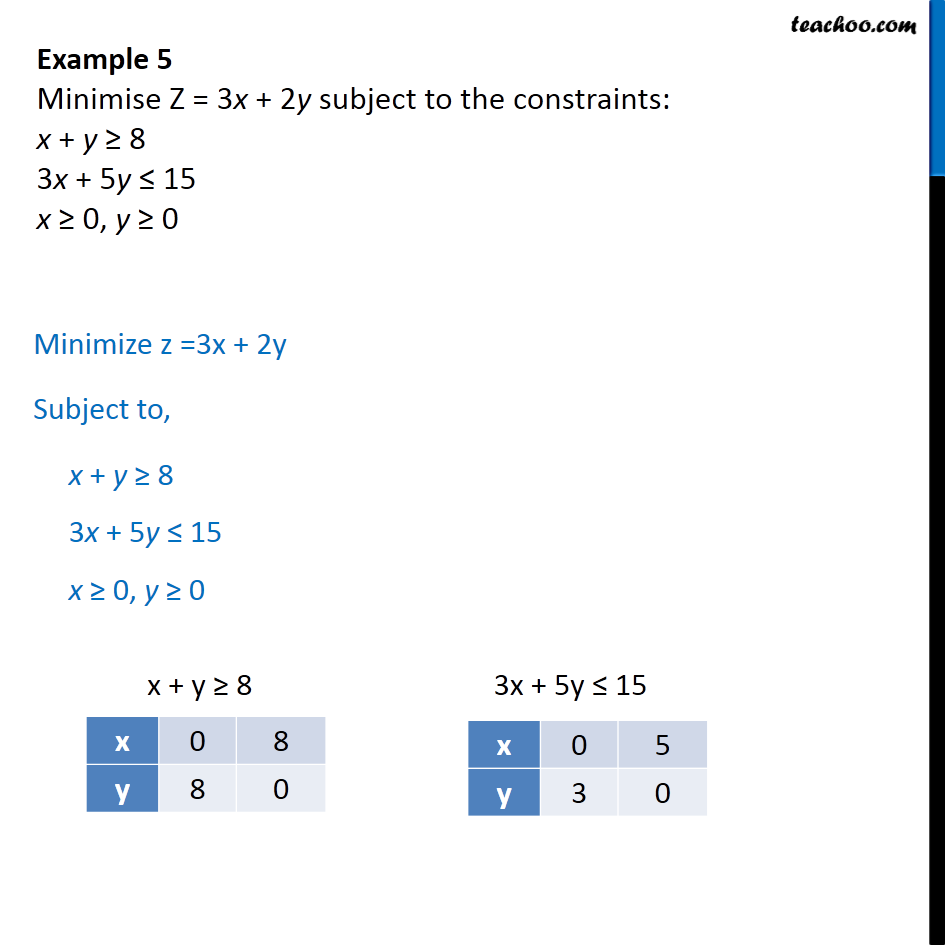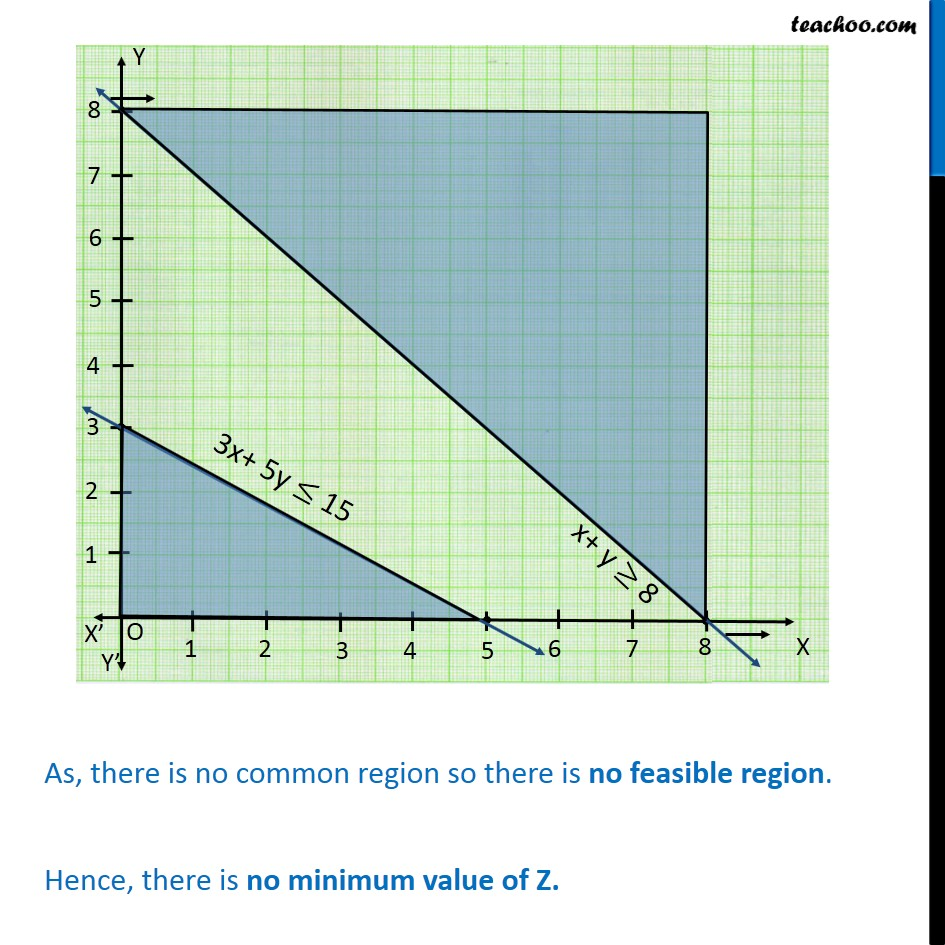Examples

Chapter 12 Class 12 Linear Programming
Serial order wiseLearn in your speed, with individual attention - Teachoo Maths 1-on-1 Class

### Transcript

Example 5 - Chapter 2 Class 12 Linear Programming - NCERT Minimise Z = 3x + 2y subject to the constraints: x + y ≥ 8 3x + 5y ≤ 15 x ≥ 0, y ≥ 0 Plotting points For x + y ≥ 8 Points are (0, 8) and (8, 0) For 3x + 5y ≤ 15 Points are (0, 3) and (5, 0) Plotting graphs of both lines And shading the relevant parts We notice that There is no common region, thus, there is no feasible region. Hence, there is no maxnimum value of Z